#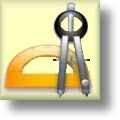Geometry Worksheets

## Coordinate Worksheets

Here is a graphic preview for all of the Coordinate Worksheets Sections. You can select different variables to customize these Coordinate Worksheets for your needs. The Coordinate Worksheets are randomly created and will never repeat so you have an endless supply of quality Coordinate Worksheets to use in the classroom or at home. We have identifying lines, rays and line segments worksheets, measurement of line segments worksheets, lines on a coordinate plane worksheets, midpoint formula worksheets, distance formula worksheets, translation, rotation, and reflection worksheets, single and four quadrant ordered pair worksheets, four quadrant graphing puzzle worksheets, standard graphing paper, single quadrant graphig paper, four quadrant graphing paper, and polar coordinate graphing paper for your use. Our Coordinate Worksheets are free to download, easy to use, and very flexible.

## Quick Link for All Coordinate Worksheets

Click the image to be taken to that Coordinate Worksheets.

##### Identify Lines, Rays, & LineSegments Worksheets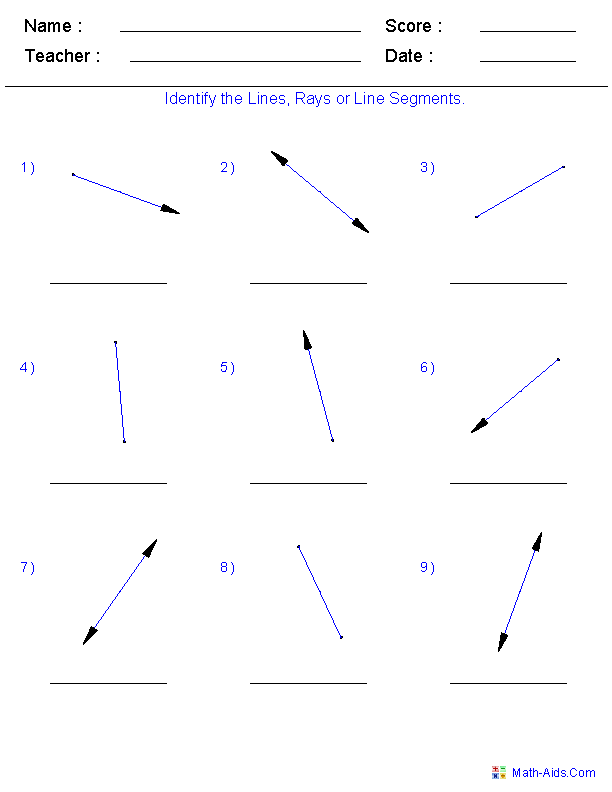##### Measuring Line Segments Coordinate Worksheets##### Equation of a Line on a PlaneCoordinate Worksheets##### Midpoint FormulaCoordinate Worksheets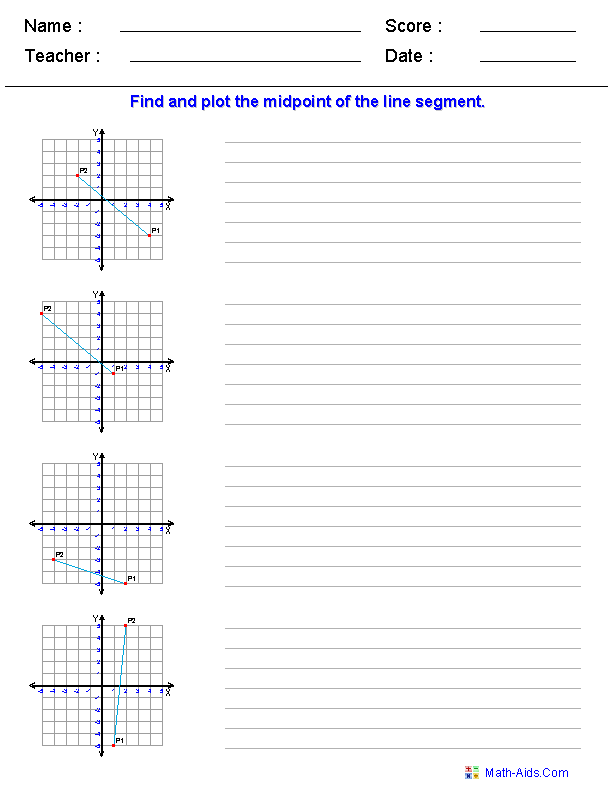##### Distance FormulaCoordinate Worksheets##### Translation, Rotation,& Reflection Worksheets##### Single Quadrant Ordered PairCoordinate Worksheets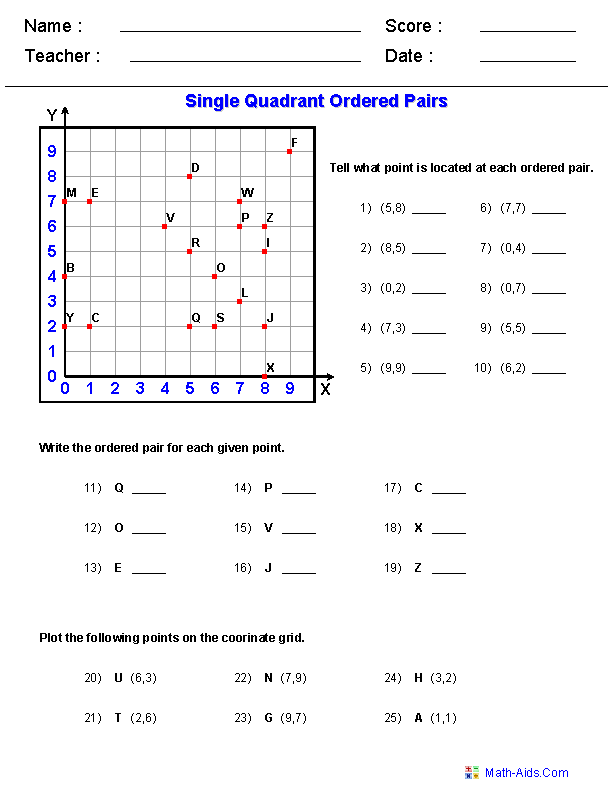##### Four Quadrant Ordered PairCoordinate Worksheets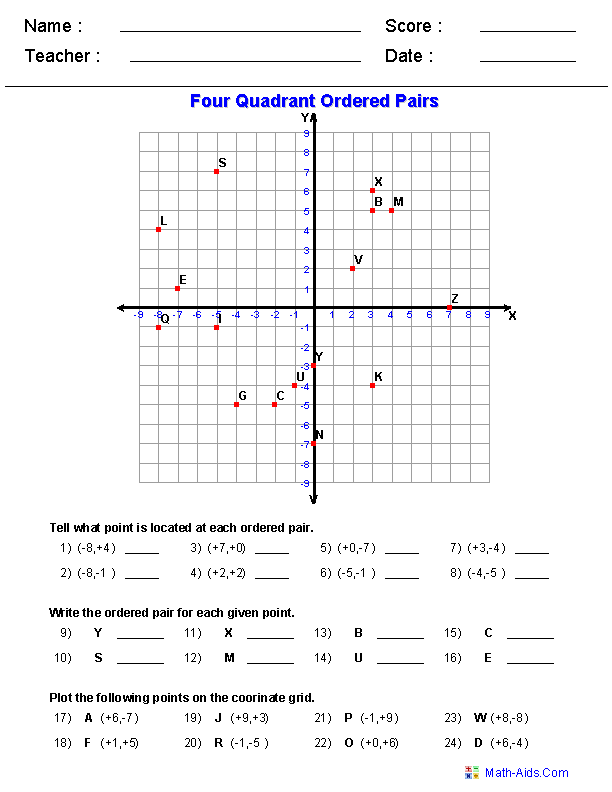##### Four Quad Graphing PuzzleCoordinate Worksheets##### Standard Graphing PaperCoordinate Worksheets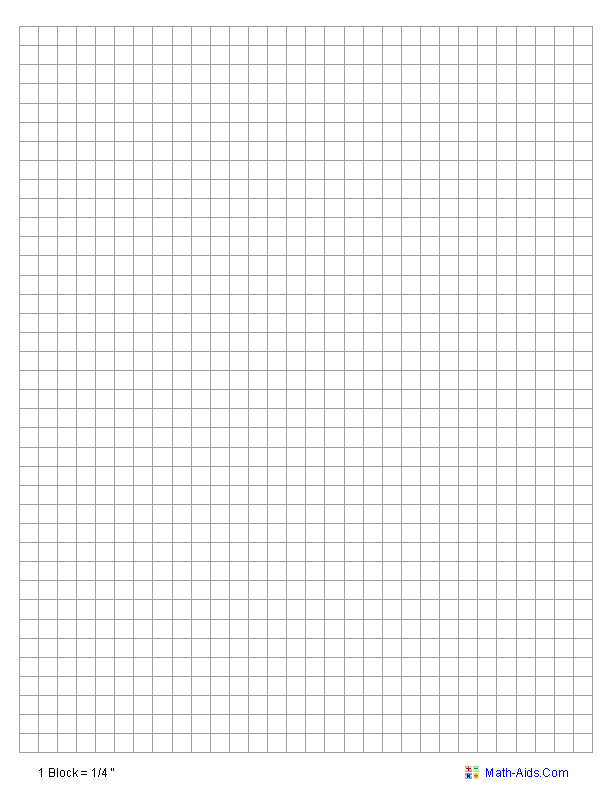##### Single Quadrant 1 Per PageCoordinate Worksheets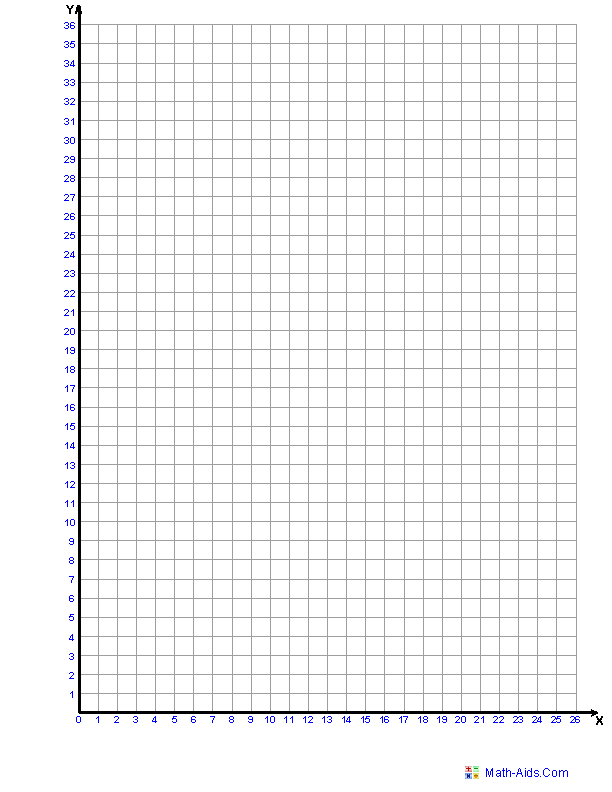##### Single Quadrant 4 Per PageCoordinate Worksheets##### Four Quadrant 1 Per PageCoordinate Worksheets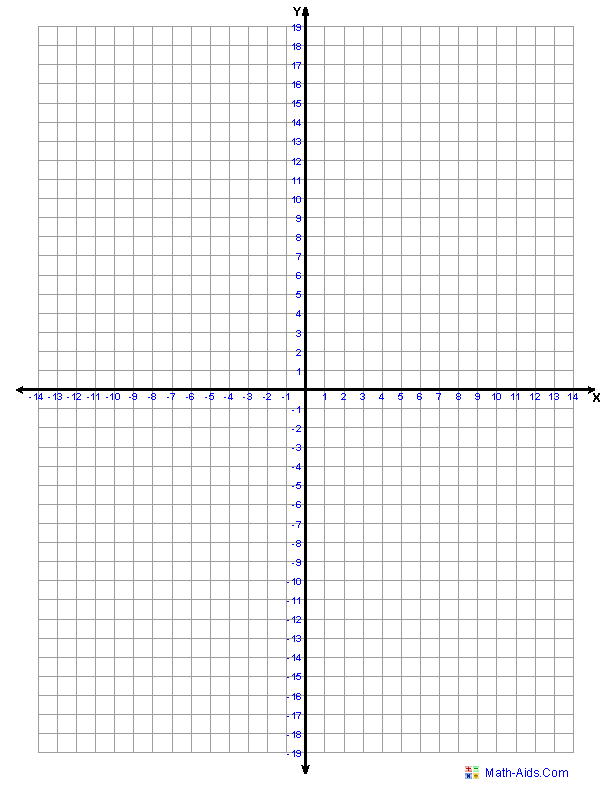##### Four Quadrant 4 Per PageCoordinate Worksheets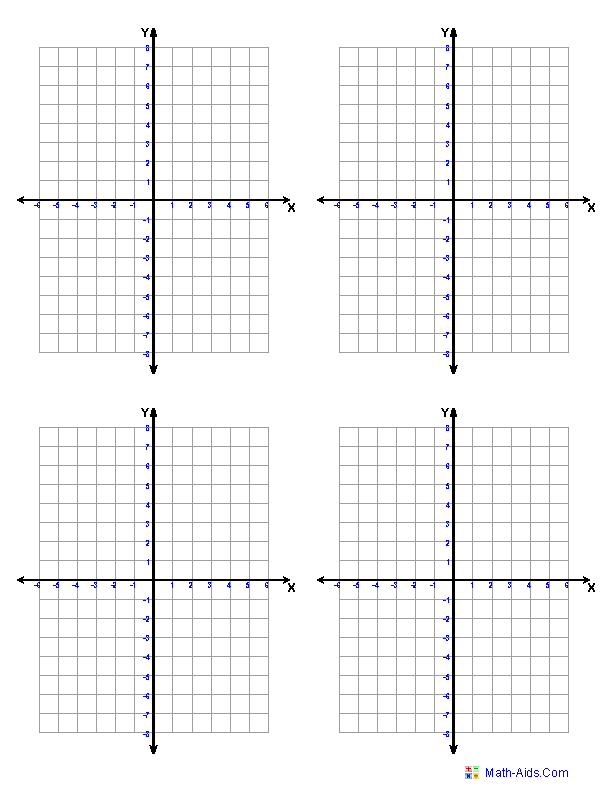##### Polar Coordinate PaperCoordinate Worksheets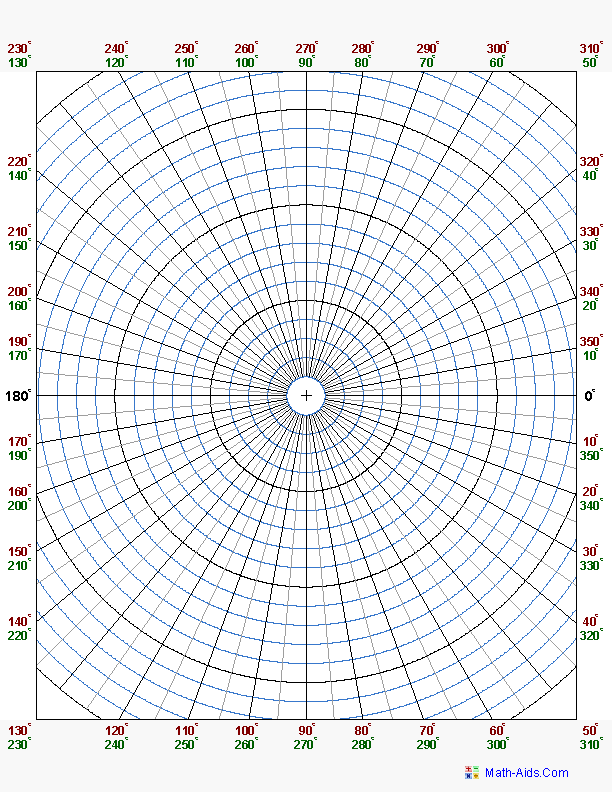Recommended Videos

## Detailed Description for All Coordinate Worksheets

Identify Lines, Rays, and Line Segments Worksheets
These Coordinate Worksheets will produce 9 problems for practicing identifying lines, rays, and line segments. These worksheets are a great resources for the 5th, 6th Grade, 7th Grade, and 8th Grade.

Measuring Line Segments Worksheets
These Coordinate Worksheets will produce problems for practicing measuring line segments. These worksheets will produce 15 problems per worksheet. These worksheets are a great resources for the 5th, 6th Grade, 7th Grade, and 8th Grade.

Equation of a Line on a Plane Worksheets
These Coordinate Worksheets will produce problems for practicing solving the equation of a line on a coordinate plane. These worksheets are a great resources for the 5th, 6th Grade, 7th Grade, and 8th Grade.

Midpoint Formula Worksheets
These Coordinate Worksheets will produce Midpoint Formula Problems for practicing solving the midpoint of a line segment on a coordinate plane. These worksheets are a great resources for the 5th, 6th Grade, 7th Grade, and 8th Grade.

Distance Formula Worksheets
These Coordinate Worksheets will produce Pythagorean Theorem Distance Problems for practicing solving distances between two sets of points on a coordinate plane. These worksheets are a great resources for the 5th, 6th Grade, 7th Grade, and 8th Grade.

Translation, Rotation, and Reflection Worksheets
These Coordinate Worksheets will produce problems for practicing identifying translation, rotation, and reflection of objects. These worksheets are a great resources for the 4th, 5th, and 6th Grade.

These Coordinate Worksheets will produce a single quadrant coordinate grid and a set of questions on ordered pairs for the student to answer. These worksheets are a great resources for the 5th, 6th Grade, 7th Grade, and 8th Grade.

These Coordinate Worksheets will produce a four quadrant coordinate grid and a set of questions on ordered pairs for the student to answer. These worksheets are a great resources for the 5th, 6th Grade, 7th Grade, and 8th Grade.

These Coordinate Worksheets will produce a four quadrant coordinate grid with a set of ordered pairs. The student will plot the points to produce the picture. These worksheets are a great resources for the 5th, 6th Grade, 7th Grade, and 8th Grade.

Standard Graphing Paper Worksheets
These Coordinate Worksheets will produce a blank page of standard graph paper for the student to use. You may vary the types of scales to be printed.

These Coordinate Worksheets will produce a single or four quadrant coordinate grid for the students to use in coordinate graphing problems. You may print 1, 2, or 4 images per page.

These Coordinate Worksheets will produce a blank page of polar coordinate graph paper for the student to use. You may vary the types of scales to be printed.